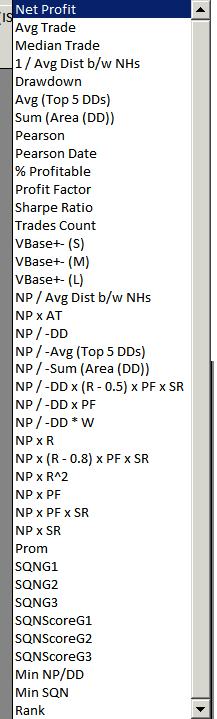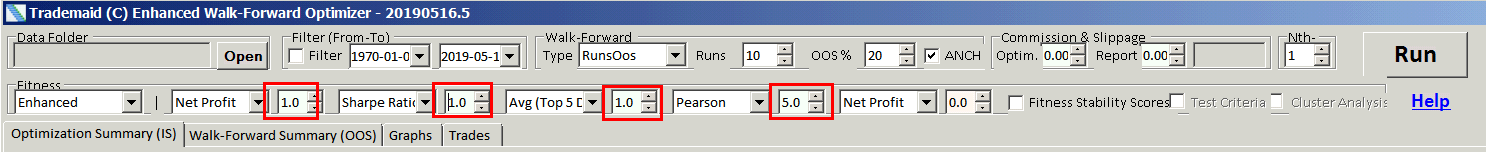The following fitness types, and combinations of them with any weighting you chose can be used.

I prefer the following.

Net profit, Net profit * ave trade, NP/DD * ave trade, Net profit/max 5 draw-downs * average trade, NP/-DD*(R-0.5)*PF*SR

Most fitness are common terms in trading, with the exceptions below.

Tradestation fitness is NP / DD * W

It calculates the Net Profit * NumWinTrades / AbsValue (Max. {Intraday} Draw-down).

Note Tradestation uses Intra day draw-down, but Tradestation WFO uses closed draw-down.

EWFO can use either. Better Trader Academy users are recommend to be consistent with WFO, and used close draw-down.

Pearson's (abbreviated to / R) is Pearson's correlation to a straight line on the equity curve.

The x axis is trade number. A perfect score would be 1.00

Pearson's date, is the as above, but the x axis is date rather than trade number.

Vbase+- is volatility based fitness. Fitness is adjusted according to the range of the market.

S is short range, M is medium range, and L is large range.

NP/-sum(area(DD)) is Netprofit / - sum of the area of the draw-down graph.

NP/-DD*(R-0.5)*PF*SR is Netprofit/draw-down *(Pearson's (see above))-0.5 * profit factor * sharpe ratio.

SQN is System quality number by Van K Tharp. This is disabled by default as its extremely CPU time consuming. This will increase your walk-forward times by 3.

See SQN for more details.### Fitness weighting

More weight can be applied to any one fitness. To have less weight, use weight <1.0